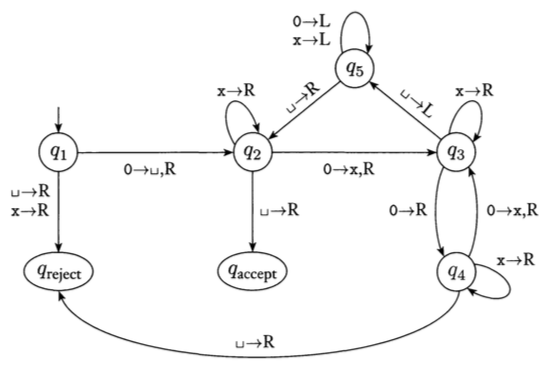Due Dates:  Oral presentations of material begin on 1 November.
Written descriptions of material presented due one week after presentation.

Topics: Turing Machines (Chapter 3).

An integral part of this class is understanding and presenting the problems assigned as homework. Everyone is expected to do all the problems, but we will take turns on who presents the problem solutions to the class (every 2-3 weeks, depending on the number of students in the class). Within a week of presenting a problem solution to the class, you must submit a written description of it, via the Blackboard system. The written solutions will be posted on the Blackboard website for the class, so, they can used by everyone to study for the exams. Since it's hard to write down answers that are concise and are easily readable by all, if you wish to improve a grade on any problem, you may resubmit it for grading.

All students enrolled should complete the following:

1. Given a state diagram for the Turing Machine in Figure 3.8:write the configurations of the machine at every state for input 0000.

2. Given a state diagram for the Turing Machine in Figure 3.8:write the configurations of the machine at every state for input 010.

3. Give a state diagram for a Turing Machine that flips every digit in the input (i.e. 0's become 1's and 1's become 0's). For example, if the input is 001101, the ending tape would have: 110010.
4. Give a state diagram for a Turing Machine that ends with two copies of the input on the tape. For example, if the input is 1001, the ending tape would have: 1001 1001.
Hint: Use an extra symbol to keep track of where you are in the string being copied.
5. Give an informal description of a Turing Machine that takes a binary number and ends with double that number on the tape. For example, if the input is 101 (or the decimal number 5), the output would be 1010 (or the decimal number 10).